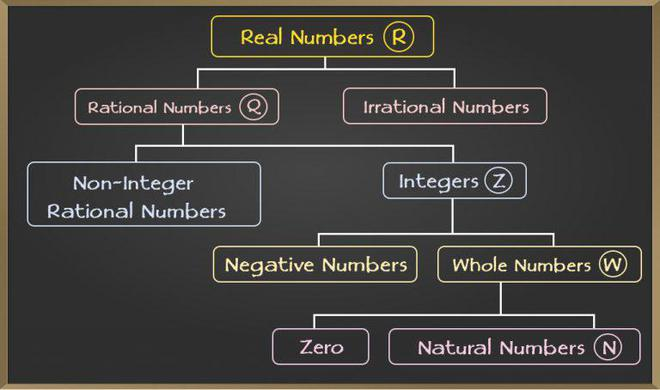GeeksforGeeks App
Open AppBrowser
Continue

# Is 0 a Natural Number?

Natural numbers are positive integers that range from 1 to infinity(∞). These numbers may be counted and are commonly used in calculations. Natural numbers are commonly denoted as “N”. We use numbers daily. They are commonly cited as numerals. We can’t count things, dates, times, money, etc., without numbers. They are sometimes used to measure, and other times used to label. The characteristics of numbers allow them to execute arithmetic operations. Both numerical and verbal delineation of these numbers is provided. For example, 8 can be written as eight, while 47 can be written as forty-seven. Students can practice writing numbers from 1 to 100 in words to find out more. Let’s learn about natural numbers before understanding 0.

## Natural Numbers

Natural numbers are a subset of real numbers that only include positive integers like 1, 2, 3, 4, 5, 6, then on while excluding negative numbers, zero, decimals, and fractions. They do not comprise negative numbers or zero. Positive integers are considered natural numbers or counting numbers, and some examples include 10, 11, 12, 13, 14, and 15,… Natural numbers, in other terms, are a collection of whole numbers other than 0. Natural numbers include 23, 39, 58, 789, 1202, and so on. It is to keep in mind that counting numbers is another name for natural numbers.

## What is 0?

0 is a number but not considered to be either a positive number or a negative number. 0 is not a positive number as it is not used for counting. For example, while counting the number of students, if there are 5 students, they will be counted as 1, 2, 3, 4, and 5. The numbers will not be counted as 0, 1, 2, 3, 4. 0 is not a negative number as there is no -ve sign attached to it. However, 0 is famously known as a neutral number.

To answer the question of whether 0 is a natural number or not? No! 0 is not a Natural number. Natural numbers, as we know, are positive integers that span from 1 to infinity. However, once we combine 0 with a positive integer like 10, 20, or the other number, we get a number. In reality, 0 may be a full number with no meaning. Below is the chart of real numbers indicating where 0 lies in the chart:## Who Invented 0?

The invention of 0 occurred in India back in 650 BC by the Indian mathematician Bramhaputra. The mathematician used dots to portray 0, known as “Shunya” in Sanskrit. He explained how 0 could be obtained by adding and subtracting. However, he was unable to explain division. It is believed that the great astronomer, Aryabhatt, first invented 0 in India.0, in this era, has so much significance that civilization would not have progressed without 0.

## Factorial of 0

The factorial of 0 is 1. The factorial of a number explains how many ways the numbers can be arranged. For example, 5! is 120. It is the multiplication of the numbers below 5, and below 5, that is, 5 × 4 × 3 × 2 × 1. However, since there is only one way 0 can be arranged, therefore, the factorial of 0 is 1. 0! is 1, and 1! is also 1.

## FAQs on Is 0 a Natural Number?

Question 1: What are the first 5 natural numbers?

Natural numbers are a subset of real numbers that only include positive integers like 1, 2, 3, 4, 5, 6, then on while excluding negative numbers, zero, decimals, and fractions. Therefore, the first 5 Natural Numbers are: 1, 2, 3, 4, and 5.

Question 2: What are the examples of Natural numbers?

The examples of natural numbers are 5, 67, 898, 3679, 91064, etc.

Question 3: What is the difference between Natural numbers and Whole numbers?

Natural numbers, which span from 1 to infinity, contain only positive integers. Whole numbers, on the opposite hand, are a mixture of zero and natural numbers that begin at 0 and terminate at an infinite value.

Question 4: Is 0 a whole number?